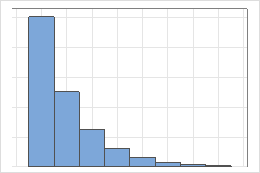# Geometric distribution

When you perform an experiment with only two outcomes, the geometric distribution is a discrete distribution that can model the number of consecutive trials that are necessary to observe the outcome of interest for the first time. The geometric distribution can also model the number of nonevents that occur before you observe the first outcome.

For example, a geometric distribution can model the number of times that you must flip a coin to obtain the first heads outcome. Similarly, for products that are built on an assembly line, the geometric distribution can model the number units that are produced before the first defective unit is produced. The following graph represents a geometric distribution with an event probability of 0.5.## What does memoryless mean?

An important property of the geometric distribution is that it is memoryless. The chance of an event does not depend on past trials. Therefore, the occurrence rate remains constant.

The memoryless property indicates that the remaining life of a component is independent of its current age. For example, random trials of a coin toss demonstrate the memoryless property. A system that has wear and tear, and thus becomes more likely to fail later in its life, is not memoryless.